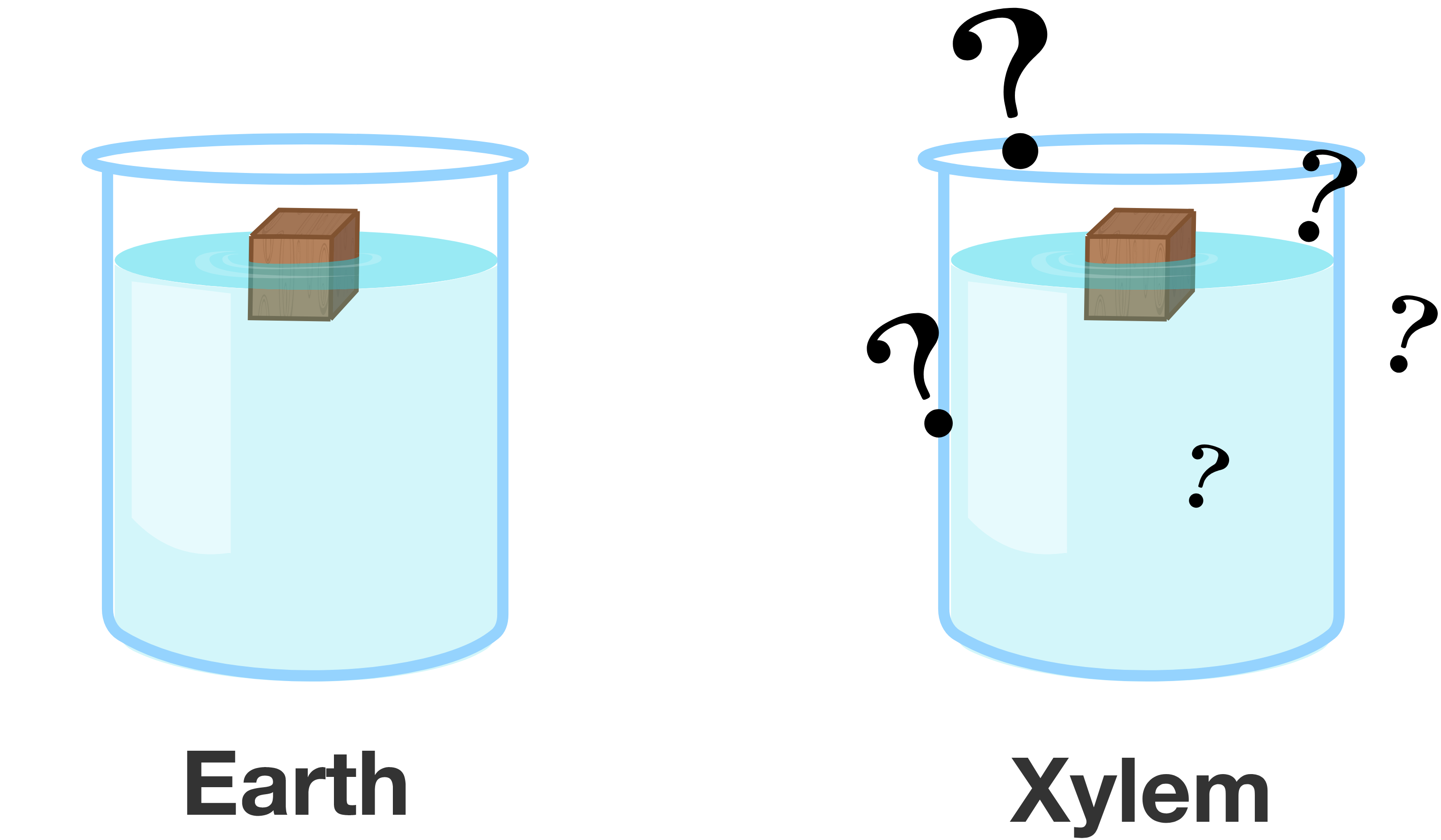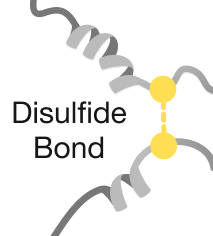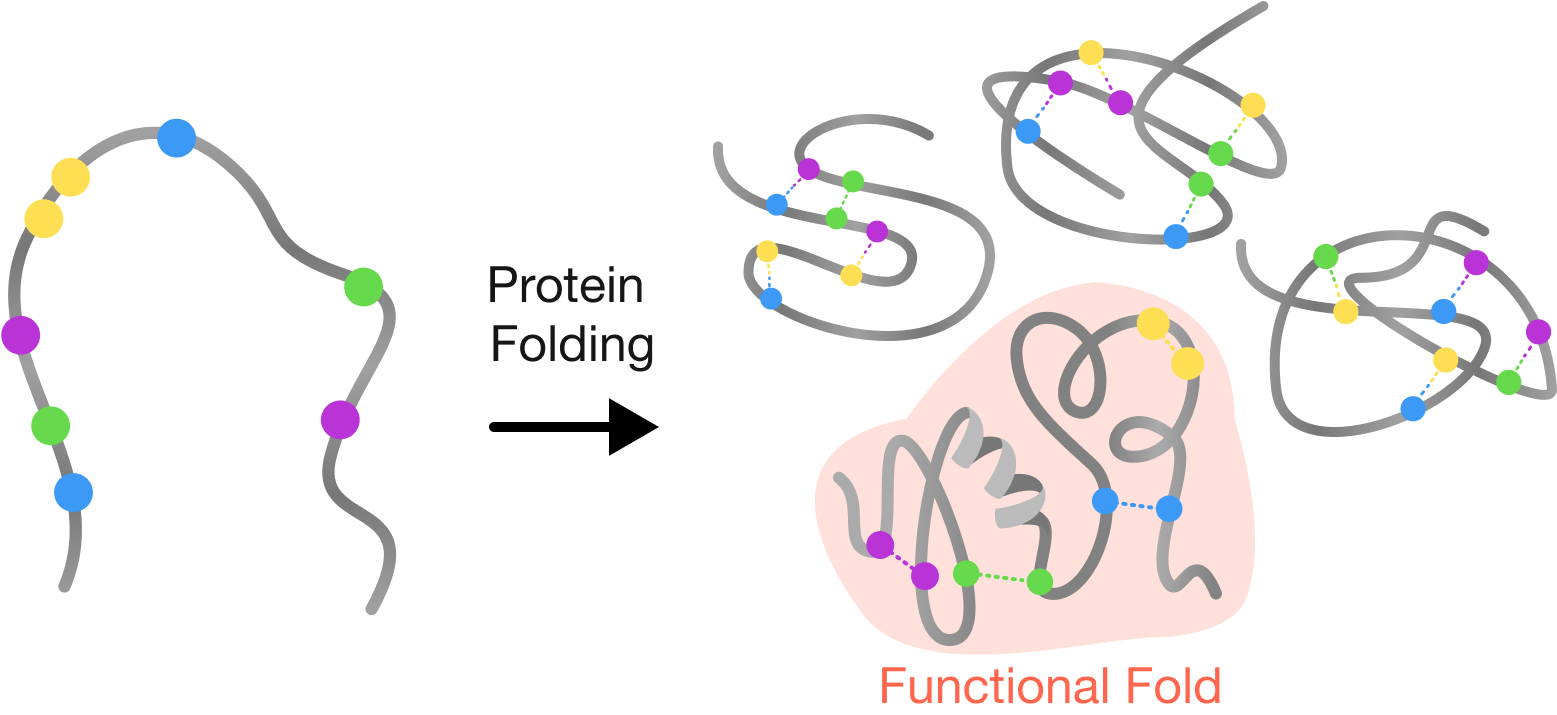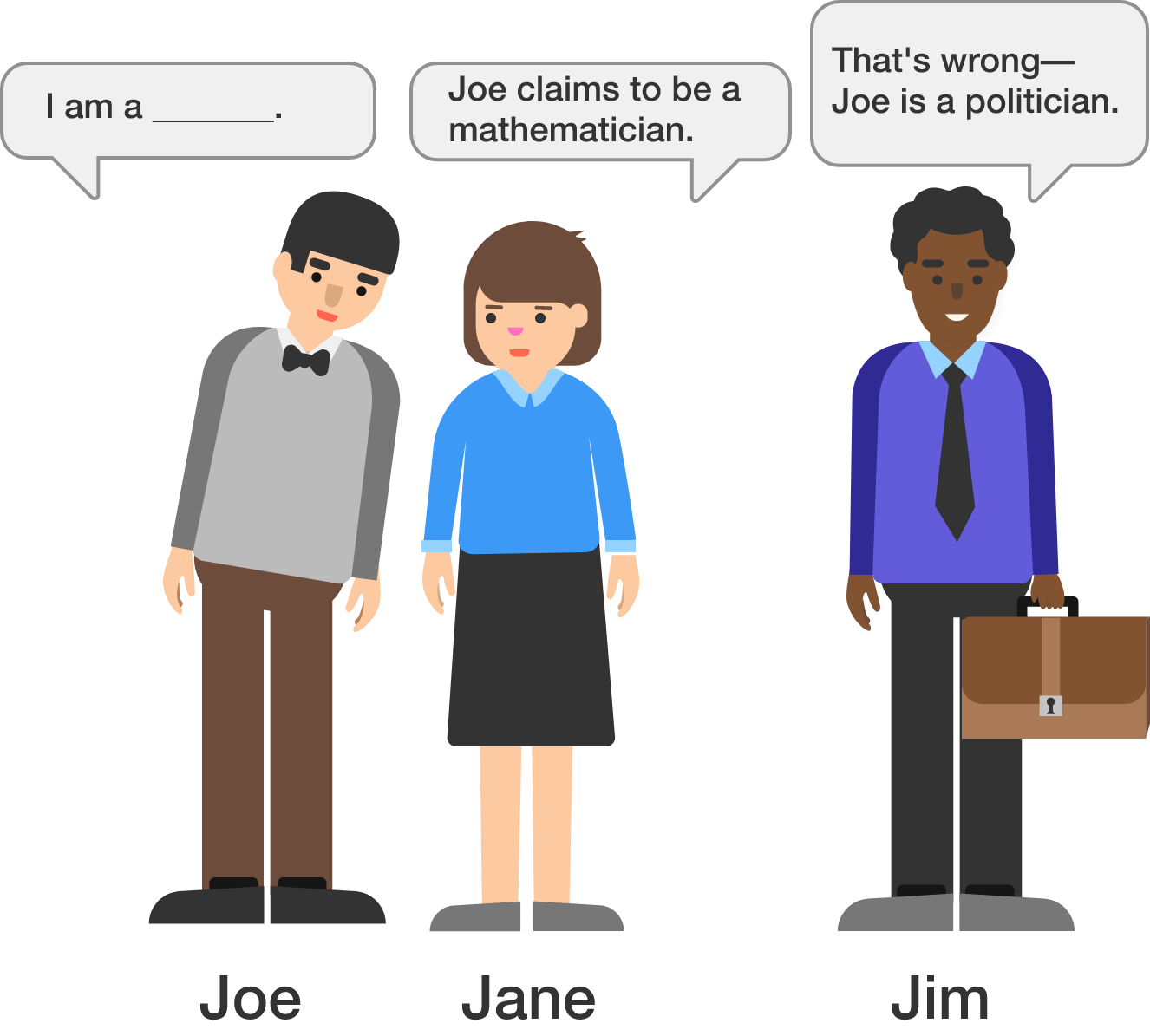# Problems of the Week

Contribute a problem

# 2018-07-16 Basic

$\begin{array} {ccccc} \large & & \color{#D61F06}F & \color{#3D99F6}A & \color{#20A900}N & \color{#69047E}G\\ \large + & & & \color{#D61F06}F& \color{#3D99F6}A & \color{#20A900}N \\ \hline \large & & 3 & 1 & 2 & 2 \end{array}$

Each letter represents a different nonzero digit. What is $G?$

A small wooden block floats in a beaker of liquid on Earth, where gravitational acceleration is roughly $\SI[per-mode=symbol]{9.8}{\meter\per\second\squared}.$ An identical wooden block floats in an identical beaker of liquid on planet Xylem, where gravitational acceleration is roughly $\SI[per-mode=symbol]{11.1}{\meter\per\second\squared}.$

How would the volume of displaced water vary in these two scenarios?$\large \left({\color{#3D99F6}3}^{23} - {\color{#3D99F6}3}^{22}\right)\left({\color{#3D99F6}3}^{24} - {\color{#3D99F6}3}^{23}\right) \left({\color{#3D99F6}3}^{25} - {\color{#3D99F6}3}^{24}\right)$

This expression can be simplified as ${\color{#D61F06}2}^{\color{#69047E}m} \times {\color{#3D99F6}3}^{\color{teal}n}$.

What is ${\color{#69047E}m} + {\color{teal}n}?$Out of the $\num{124}$ amino acids that make up the ribonuclease enzyme, there are $\num{8}$ cysteines. These amino acids can come together like velcro to form strong bonds that hold a protein into one folded configuration. A cysteine can form a bond with any other cysteine, and every cysteine is always paired; so the enzyme has a total of four bonds.

Only one arrangement of bonds will yield a correctly folded and functional ribonuclease enzyme capable of chopping up RNA molecules. In most cases, this folding process does not depend on the order that the bonds are formed.

If each protein randomly folds into one arrangement, what fraction of enzymes will be functional?In the country Logicala, everyone is either a politician or a mathematician. Mathematicians always tell the truth and politicians always tell lies.

One day, three people from Logicala meet: Joe, Jane, and Jim.

• Joe whispers to Jane, telling her either “I am a politician” or “I am a mathematician.”
• Jane tells Jim, “Joe claims to be a mathematician.”
• Jim replies, “That's wrong—Joe is a politician.”

How many of the three people are mathematicians?×

Problem Loading...

Note Loading...

Set Loading...